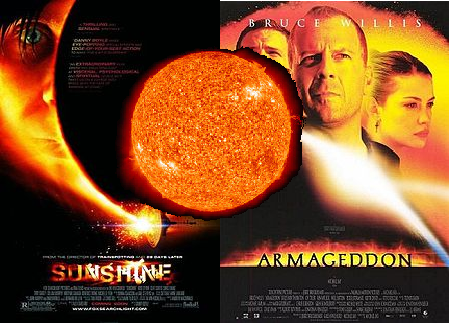<-- .. title: When will the Earth fall into the Sun? .. date: 2012-11-29 22:58:00 .. category: old .. slug: when-will-the-earth-fall-into-the-sun- .. author: Brian .. has_math: true -->The time I spent making this poster could have been spent doing research

Since December 2012 is coming up, I thought I'd help the Mayans out with a look at a possible end of the world scenario. (I know, it's not Earth Day yet, but we at the Virtuosi can only go so long without fantasizing about wanton destruction.) As the Earth zips around the Sun, it moves through the [heliosphere](http://en.wikipedia.org/wiki/Heliosphere), which is a collection of charged particles emitted by the Sun. Like any other fluid, this will exert drag on the Earth, slowly causing it to spiral into the Sun. Eventually, it will burn in a blaze of glory, in a bad-action-movie combination of Sunshine meets Armageddon. Before I get started, let me preface this by saying that I have no idea what the hell I'm talking about. But, in the spirit of being an arrogant physicist, I'm going to go ahead and make some back-of-the-envelope calculations, and expect that this post will be accurate to within a few orders of magnitude. Well, how long will the Earth rotate around the Sun before drag from the heliosphere stops it? This seems like a problem for fluid dynamics. How do we calculate what the drag is on the Earth? Rather than solve the fluid dynamics equations, let's make some arguments based on dimensional analysis. What can the drag of the Earth depend on? It certainly depends on the speed of the Earth v -- if an object isn't moving, there can't be any drag. We also expect that a wider object feels more drag, so the drag force should depend on the radius of the Earth R. Finally, the density of the heliosphere might have something to do with it. If we fudge around with these, we see that there is only 1 combination that gives units of force: $$F_{drag} \sim \rho v^2 R^2$$ Now that we have the force, the energy dissipated from the Earth to the heliosphere after moving a distance $d$ is $E_\textrm{lost} = F\times d$. If the Earth moves with speed v for time t, then we can write $E_\textrm{lost} = F v t$. So we can get an idea of the time scale over which the Earth starts to fall into the Sun by taking $E_\textrm{lost} = E_\textrm{Earth} \sim 1/2 M_\textrm{Earth} v^2$. Rearranging and dropping factors of 1/2 gives $$T_\textrm{Earth burns} \sim M_{Earth} v^2 / (F_{drag}\times v) \\ \qquad \sim M_{Earth} / (\rho R^2 v)$$ Using the velocity of the Earth as $2\pi \times 1 \mbox{Astronomical unit/year}$, Googlin' for some numbers, and taking the [density of the heliosphere](http://web.mit.edu/space/www/helio.review/axford.suess.html) to be $10^{-23}$ g/cc we get... $$T \approx 10^{19} \textrm{ years}$$ Looks like this won't be the cause of the Mayan apocalypse. (By comparison, the [Sun will burnout](http://en.wikipedia.org/wiki/Sun#Life_cycle) after only $\sim10^9$ years.)